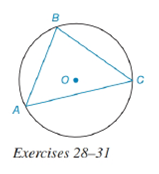Chapter 6.4, Problem 27E### Elementary Geometry for College St...

6th Edition
Daniel C. Alexander + 1 other
ISBN: 9781285195698

#### Solutions

Chapter
Section### Elementary Geometry for College St...

6th Edition
Daniel C. Alexander + 1 other
ISBN: 9781285195698
Textbook Problem
1 views

# Given circle O with m B C ⌢ = 120 ° , and m A C ⌢ = 130 ° .a) Which angle of triangle A B C is smallest?b) Which side of triangle A B C is nearest point O ?To determine

(a)

To find:

The smallest angle of triangle ABC.

Explanation

Given:

Circle O with mBC=120°, and mAC=130°. The figure given below

Property Used:

The measure of an inscribed angle of a circle is half the measure of its intercepted arc. Consider the figure below, let the inscribed angle be 2

Then m2=12mHJ and generally HKKJ.

Calculation:

Since, the measure of an inscribed angle of a circle is half the measure of its intercepted arc and from the given figure

To determine

(b)

To find:

The side of triangle ABC that is nearest point O.

### Still sussing out bartleby?

Check out a sample textbook solution.

See a sample solution

#### The Solution to Your Study Problems

Bartleby provides explanations to thousands of textbook problems written by our experts, many with advanced degrees!

Get Started

#### Find more solutions based on key concepts